# Joint Peristimulus Time Histogram (JPSTH)

Note: We're happy to freely distribute the source code for our programs. Currently we use MATLAB (augmented, occasionally, with compiled code from other languages such as FORTRAN). For additional information or to request our source code, please send an email to software@mulab.physiol.upenn.edu.

In this section we will describe another crosscorrelation analytical tool, called the Joint Peristimulus Time Histogram, or JPSTH. We assume that you are familiar with the basic concepts of neural spike trains (as discussed in our Introduction and Background Material section) and crosscorrelation (discussed in the Crosscorrelation section).

The simple crosscorrelogram described above is "one-dimensional" -- it collapses the data across the duration of the stimulus and only reports correlations based on averages. In other words, a crosscorrelogram may tell you that there was a tendency for neuron B to fire about 4 ms after neuron A, but it doesn't indicate if this tendency was only near the beginning of the stimulus, or if it was stronger near the end of the stimulus, or whether the tendency was relatively constant throughout the time of the stimulus. So, if the correlation was changing over the course of the stimulus -- i.e., if it was "dynamic" -- this fact cannot be reported by the one-dimensional crosscorrelogram.

The JPSTH is a way of investigating the dynamics of correlation, as well as providing a bit more sensitive means of detecting correlation. An example of a JPSTH is shown in Figure 11. A description of the features of the JPSTH follows. The most important feature, the matrix, is discussed first and is described in terms of its construction.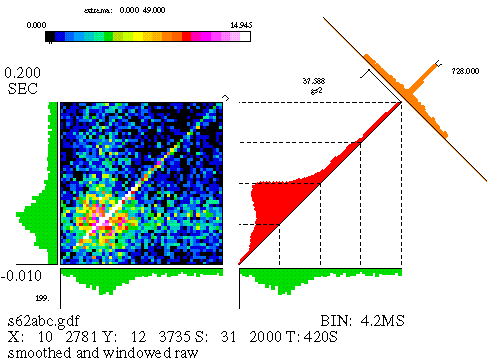Figure 11

We assume that the experiment delivered a certain number of stimuli to a pair of neurons, and that the spikes of two cells were simultaneously recorded. The JPSTH matrix is shown on the left-hand side of Figure 11; it consists of square bins, of area (dt) squared, where dt is the bin size used for the individual spike trains' PSTH. The matrix is computed by taking each stimulus trial segment (aligned with the stimulus marker) and plotting the spikes of each cell, one cell on the horizontal and one on the vertical. (See Figure 12.) The bins of the matrix that contain a coincidence of spikes are incremented. For example, if there's a spike from neuron A at time x, and a spike from neuron B at time y, we add one count to the matrix bin containing the point (x,y). Repeating this process for each stimulus trial gradually produces one neuron's PSTH along the horizontal axis and the other along the vertical axis, as well as the "raw" JPSTH matrix. Generally the matrix values are either color-coded or gray-scaled; if we used height instead, we would need three dimensions to depict the matrix, and getting the perspective right for this kind of figure isn't trivial.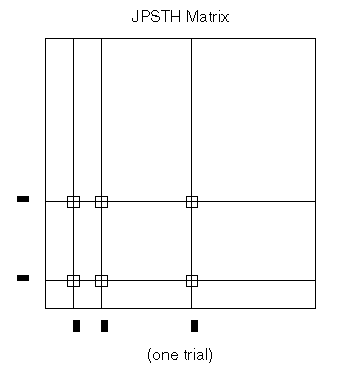Figure 12

There are other computations that we need to perform on the matrix in order to yield a more useful form, but first we'll describe the other components of the JPSTH. Figure 11 is reproduced in a smaller size below.

We are most interested in the little strip of bins running along the main diagonal of the matrix (or a small distance on either side of the main diagonal). These bins encompass most of the temporal range of physiological interactions; the areas in the upper left and lower right corner represent spike pairs which were separated by a much larger amount of time. Hence, the paradiagonal bins are of great importance and we plot them separately, as seen to the immediate right of the matrix in Figure 11. This histogram, which runs along the diagonal of the square area beside the matrix, is called the "coincidence" histogram, since it represents coincident or near-coincident firing of the cells (which is time-locked to the stimulus). The coincidence histogram is computed using slightly different bins than were used for the matrix; we use rectangular bins oriented along a 45 degree angle with respect to the horizontal. Details aside, the coincidence histogram is basically just a cross-section of the matrix along the strip indicated by the marker appearing in the top right corner of the matrix.

The other component of the JPSTH is the histogram appearing at a right angle to the coincidence histogram; this histogram represents the one-dimensional crosscorrelogram and is computed by summing the bins along the appropriate paradiagonal.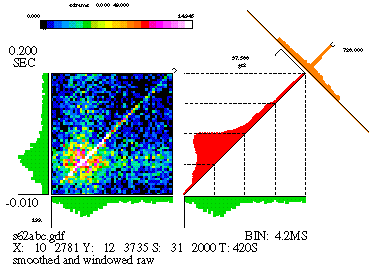It's an easy way to look at the correlation, averaged over the stimulus duration. (Note: computation of the crosscorrelogram is complicated by the fact that the paradiagonals are not the same length; thus, they must either be extended or normalized to compute a fair crosscorrelogram.)

The text appearing in the figure either describes the data or indicates scale values. (The particular arrangement of text varies with each different laboratory's version of the JPSTH.) The text along the bottom identifies the number of spikes in each train (e.g., the horizontal, or 'X' axis, cell id. number 10 has 2781 spikes); the stimulus marker, 'S', is id. number 31 and was repeated 2000 times. On the left side, we see that the analysis window began at -0.01 seconds (with respect to the stimulus marker) and ends at .2 seconds, making the window 210 milliseconds long. Scale values appear for each component of the figure (for example, the PSTHs), though they're probably too small to make out in the reduced form presented here. Finally, at the top is the color scale for the matrix, replaced by a gray scale for B&W reproductions.

The data used for this figure is from a simulation. There's an excitatory synapse from cell 10 (the 'X' axis) to cell 12 ('Y'), and this clearly shows up in the large values seen along the diagonal of the matrix, as well as in the peak of the crosscorrelogram. But there's more to this simulation than just that: the strength of the synapse was modulated, in that the synaptic strength quickly rises when the stimulus begins and slowly decays over time. We'll see later how the JPSTH can pull this information out of the data. The raw matrix, as well as the coincidence histogram produced from the raw matrix, is difficult to interpret since it is heavily influenced by stimulus-locked firing rate correlations (see below).

Now let's return to the raw matrix. It gives us a picture of the correlation between 2 spike trains, as seen by a neuron receiving input from both. Neurophysiologically, that's actually the most relevant thing -- the "correction" and statistical formulas that we apply to the data are not very likely to be replicated by neurons in real nervous systems. However, if our goal is to study the functional connectivity of the cells in question, we need to make some further computations.

It is very important that we remove the correlations due to co-stimulation. As discussed in the section on crosscorrelation, when we experimentally stimulate a pair of cells we generally elevate their firing rate; and since we're stimulating the cells at the same time, this will cause the spike trains of the cells to co-vary. The cells' spike trains are thus correlated -- but we'd like to remove this experimentally-induced correlation for further data analysis. To this end, the "shift predictor" is computed; for the JPSTH, this is a matrix constructed exactly like the "raw" matrix but with a relative shift in the spike trains. For example, we may shift one spike train a distance of one stimulus trial to the left, and under these circumstances we are computing the matrix by comparing the n th trial of one spike train with the (n+1) th trial of another. Shifted spike trains retain the firing rates of the spike trains but destroy any physiologically induced correlation (except as produced by the stimulus), since such correlation acts on relatively small time scales and is not expected to endure across different stimulus trials. The shift predictor is subtracted bin by bin from the raw matrix, thereby effectively eliminating the time-locked stimulus-induced covariation. (Note: In practice, you get a better and less noisier shift predictor by averaging over all possible shifts. Computationally, this is the same as taking the cross-product of the 2 neurons' PSTHs.)

The "corrected" JPSTH matrix is displayed in Figure 13. Note that the coincidence histogram and crosscorrelogram that are displayed are now for the corrected matrix, not the raw. The final form of the corrected matrix is obtained by subtracting bin by bin the shift predictor from the raw matrix, as explained above, and then dividing, again bin by bin, by the product of the individual neurons' PSTH standard deviations. (The PSTH standard deviation is computed by taking the standard deviation of the set of PSTH bin counts.) The corrected matrix counts become correlation coefficients, and have a value between -1 and 1. Converting the matrix bin counts into correlation coefficients facilitates comparison of matrices between different data sets.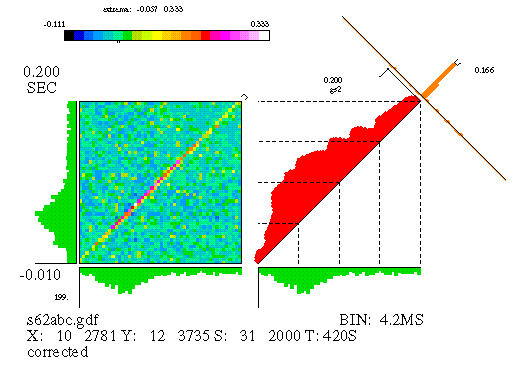Figure 13

The corrected matrix indicates areas of excess or deficit correlation over the time course of the stimulus. Look at the coincidence histogram; you might want to compare it with that reported by the raw matrix, in Figure 11. As noted above, the simulation that produced this data maintained a dynamic relationship between the 2 neurons, described by an initial rapid rise and subsequent slow decay. The corrected coincidence histogram now reveals this feature. Also note that the "background" has disappeared from the matrix and the crosscorrelogram.

However, we're still not quite done. What about the significance of the results? Unfortunately, extreme bin values in the JPSTH matrix may simply be due to chance, and statistical tests for the significance of these values are in order. One such test is quite similar to that which was explained in the section on crosscorrelation in this tutorial: it assumes a Poisson distribution for the bin counts of the raw matrix, with the shift predictor count as mean and (since we're assuming a Poisson distribution) the square root of the shift predictor as the standard deviation. Note that this test is applied to each matrix bin, using the corresponding bin of the shift predictor to estimate the distribution parameter (fortunately, the Poisson distribution has only one parameter). The probability of observing a bin count equal to or greater than the raw value can be computed. If this probability is low -- say, p < 0.01 -- then that bin's count can be considered highly unlikely to have been produced by 2 independent spike trains.

Another test has been developed for assessing significance of the JPSTH bin values. We're not going to explain this procedure in detail, so we'll be concise in our description of it. Basically, the test uses the data to compute an expected distribution of bin counts in the JPSTH, under the assumption that the spike trains are independent. Calculation of the distributions employs a counting process, with coincidences assumed to be binary (either 1 or 0, meaning that we don't expect to observe more than one spike-to-spike coincidence in any matrix bin for any given stimulus trial); the process derives something similar to the hypergeometric distribution (which is often used for computing the probability distribution of a sampling procedure without replacement). The logarithm of the derived probability is called "surprise" and this is the number that's reported in the surprise JPSTH matrix, as shown in Figure 14. This test clearly indicates the statistical significance of the correlation; the white areas of the matrix show which bins have counts that were highly unlikely to be due to chance. (Note: Whether the term "surprise" is descriptive and appropriate is regrettably debatable.)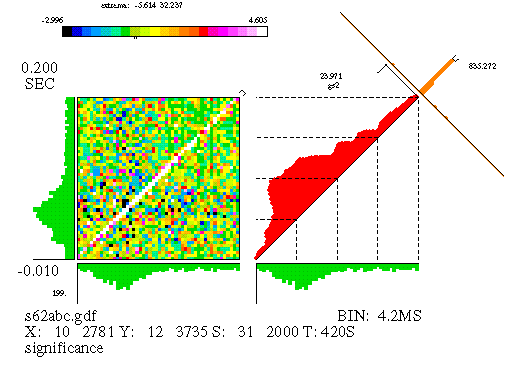Figure 14

We now have a tool -- our choice of 2, in fact -- to determine the statistical significance of the JPSTH matrix bin counts. (For stationary data, the 2 tests would probably show strong agreement; it is not known, however, whether they would give identical results for nonstationary data.) Unfortunately we have to keep in mind that both tests are performed "binwise", i.e., on each bin. One cannot conclude, on the basis of a small number of statistical significant bin counts, that the two spike trains being analyzed are dependent. This is quite similar to the problem generated by the statistical tests for the crosscorrelogram, except the danger is exacerbated here since the JPSTH has, generally, many more bins -- and therefore many more false positives, depending on what value of "p", or statistical significance level, was selected. As in the crosscorrelogram, one decides the dependence or independence of the spike trains by looking for structure in the statistical test results; if there is a relatively large number of bins, forming a contiguous region, in which the tests reveal significance, then one can safely reject the hypothesis that the spike trains are independent.

The power of the JPSTH is evident in the amount of information it supplies compared with the crosscorrelogram. Crosscorrelograms may permit you to infer dependence of the spike trains under analysis, and gives you an indication of the nature of the relationship on average . The JPSTH gives you not only this ability, but also displays the stimulus-related dynamics of the relationship. As many scientists know, under certain circumstances averages do not provide sufficient information, and under some circumstances an average can even be misleading. The temporal information which is given by the two-dimensional nature of the JPSTH can thus be quite important in studying neural connectivity.

Using the JPSTH (or the crosscorrelogram, for that matter) on electrophysiological data, and then drawing inferences accordingly, is exhilirating particularly when the data allow some intriguing conclusions. However, there is an inevitable uneasiness with using tools by which conclusions are inferred and not demonstrated. Our lab, among others, have used simulations to test the accuracy of the JPSTH; in such simulations, the researcher knows the properties of the neurons under study as well as their relationships, and can in fact configure the cells in any desired way. In these tests the JPSTH has been found to be generally quite accurate, as you can see from the example we used here. The unfortunate exceptions to this rule are presented next.

### Caveats of the JPSTH

The JPSTH, like everything else, has its limitations. The major caveat is applying the statistical calculations on data which is nonstationary, or in misinterpreting the corrected matrix under such circumstances. This problem is quite similar to that of the crosscorrelogram, as was covered under the heading "Caveats" in the section on crosscorrelation in this tutorial. (See Nonstationarities .)

However, recent advances in the JPSTH have identified some promising approaches to mitigating the nonstationarity problem. One approach uses the marginal distributions of the raw JPSTH matrix instead of the PSTHs for correction purposes; another approach is based on the spike counts of each neuron for each stimulus trial. Both these approaches are in the developmental stage.

Another problem, as discussed in the crosscorrelation section, is the difficulty in interpreting data of small quantity. If you don't have very many spikes to analyze -- or, in other words, if the sample size is small -- then inferences are difficult to make. (And difficult to statistically support.) As you might expect, this problem is quite a bit more onerous for the JPSTH than the crosscorrelogram, since the JPSTH analyzes data throughout the stimulus duration, whereas the crosscorrelogram averages over this time period. That means that you generally need quite a few more spikes to use the JPSTH reliably than you do for the crosscorrelogram.

Kyle Kirkland

Back Home